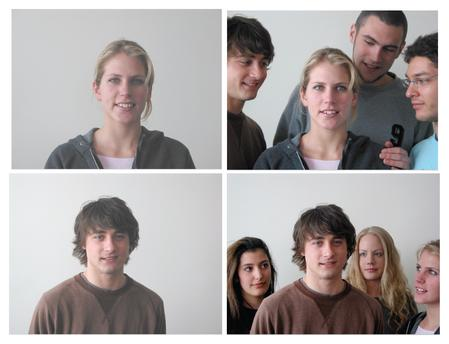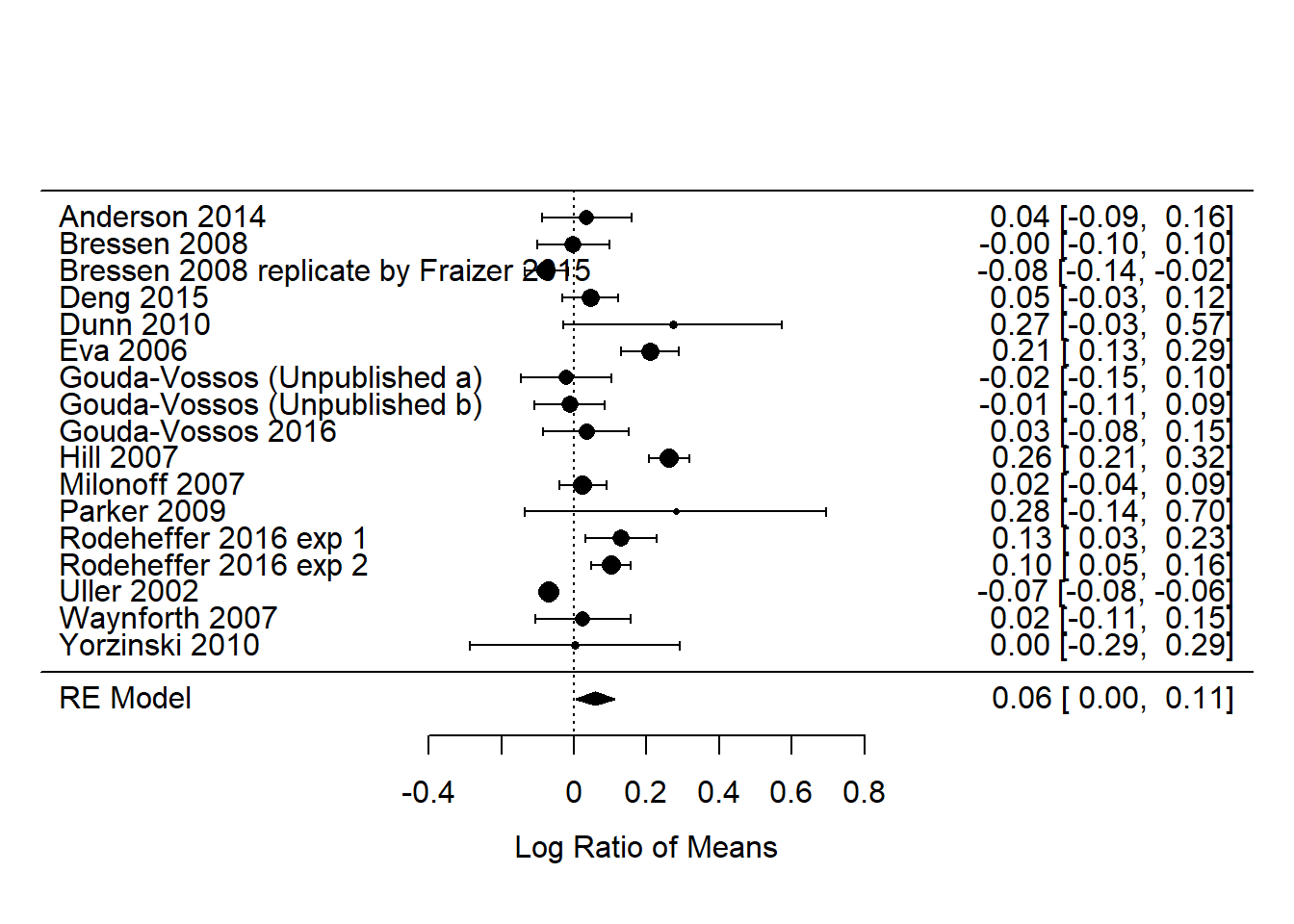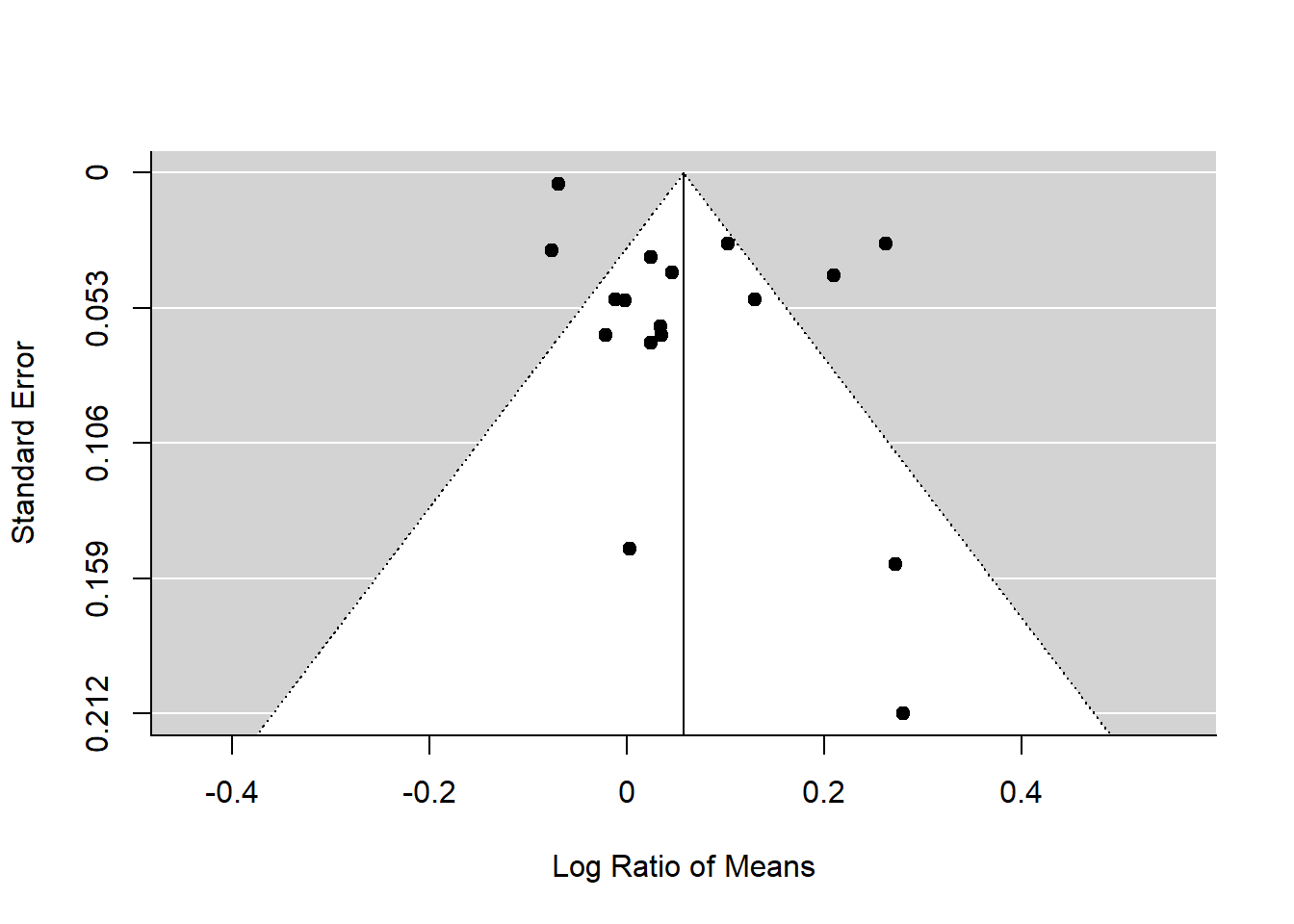## Introduction to meta-analysis

As the number of scientific studies continues to grow exponentially, so does the opportunity to gain insights on a specific hypothesis using data from a large number different studies. Literature reviews are useful for providing a synthesis on the current understanding of a particular research topic, but are largely qualitative in nature and are unable to quantitatively assess conflicting results from different studies. Meta-analysis provides a statistical framework for combining and comparing different studies to test a specific research hypothesis.

In this SEEC Stats Toolbox Seminar you will find out about:

1. how to conduct a rigorous meta-analysis,
2. learn what effect sizes are in meta-analyses,
3. find out about useful R packages and tools for conducting meta-analyses.

To find out more, download/clone all the Toolbox files from GitHub here, or get the slides, video and code separately.

The example used for this Toolbox is from Gouda-Vossos et al. (2018) and deals with mate choice in humans. The authors did a meta-analysis of experiments in which the “attractiveness” of a person was rated before and after a treatment, which involved either “addition” or “augmentation” designs. In this example we are only going to look at their experiment (i), which was an “addition” experiment in which the attractiveness of females was rated when they were alone (the “control”) and then when they were surrounded by a number of men (the “treatment”).An example of a “mate choice experiment” in humans. From Dunn & Doria (2010).

In this Markdown document I provide a little bit of code to help get you started with doing a basic meta-anlysis. You will find out how to:

• Calculate effect sizes
• Run fixed-effect and random-effects meta-analytic models
• Run a meta-regression
• Produce forest plots
• Check for publication bias

## Getting started in R

library(metafor) #Install this package first if you do not have it
head(dat)
##                             First_Author Mean_without SD_without
## 1                          Anderson 2014        3.040      1.550
## 2                           Bressen 2008        5.290      1.710
## 3 Bressen 2008 replicate by Fraizer 2015        4.760      1.710
## 4                              Deng 2015        3.659      0.980
## 5                              Dunn 2010        5.080      4.136
## 6                               Eva 2006        2.960      0.580
##   Number_without Mean_with SD_with Number_with  Design No_of_Stim
## 1            123      3.15    1.52         121  Within          5
## 2             52      5.28    1.50         156 Between         12
## 3            263      4.41    1.50         263 between         12
## 4             90      3.83    0.98          90  Within         50
## 5             40      6.67    3.54          40 Between         12
## 6             38      3.65    0.55          38 Between         10

## Calculate effect sizes

We use the log of the response ratio (lnRR), which is the mean of the treatment (M_T) divided by the mean of the control (M_C)

dat = escalc(measure="ROM", m1i=Mean_with, m2i=Mean_without, sd1i=SD_with, sd2i=SD_without, n1i=Number_with,
n2i=Number_without, data=dat)
head(dat)
##                             First_Author Mean_without SD_without
## 1                          Anderson 2014        3.040      1.550
## 2                           Bressen 2008        5.290      1.710
## 3 Bressen 2008 replicate by Fraizer 2015        4.760      1.710
## 4                              Deng 2015        3.659      0.980
## 5                              Dunn 2010        5.080      4.136
## 6                               Eva 2006        2.960      0.580
##   Number_without Mean_with SD_with Number_with  Design No_of_Stim      yi
## 1            123      3.15    1.52         121  Within          5  0.0355
## 2             52      5.28    1.50         156 Between         12 -0.0019
## 3            263      4.41    1.50         263 between         12 -0.0764
## 4             90      3.83    0.98          90  Within         50  0.0457
## 5             40      6.67    3.54          40 Between         12  0.2723
## 6             38      3.65    0.55          38 Between         10  0.2095
##       vi
## 1 0.0040
## 2 0.0025
## 3 0.0009
## 4 0.0015
## 5 0.0236
## 6 0.0016

## Fixed-effect and random-effects meta-analytic models for (i)

In the paper, the authors present the results of a random-effects meta-analytic model, which is more conservative and accounts for non-independence of effect sizes (i.e. assumes the studies do not come from the same population, which is a realistic assumption).

The fixed-effect model is merely provided for comparison.

#Random-effects model
model_i_re = rma(yi, vi, data=dat, method='REML')
summary(model_i_re)
##
## Random-Effects Model (k = 17; tau^2 estimator: REML)
##
##   logLik  deviance       AIC       BIC      AICc
##  12.2117  -24.4233  -20.4233  -18.8781  -19.5002
##
## tau^2 (estimated amount of total heterogeneity): 0.0091 (SE = 0.0043)
## tau (square root of estimated tau^2 value):      0.0952
## I^2 (total heterogeneity / total variability):   89.50%
## H^2 (total variability / sampling variability):  9.53
##
## Test for Heterogeneity:
## Q(df = 16) = 254.5417, p-val < .0001
##
## Model Results:
##
## estimate      se    zval    pval   ci.lb   ci.ub
##   0.0575  0.0272  2.1099  0.0349  0.0041  0.1109  *
##
## ---
## Signif. codes:  0 '***' 0.001 '**' 0.01 '*' 0.05 '.' 0.1 ' ' 1
#Fixed-effect model
model_i_fe = rma(yi, vi, data=dat, method='FE')
summary(model_i_fe)
##
## Fixed-Effects Model (k = 17)
##
##   logLik  deviance       AIC       BIC      AICc
## -91.6087  254.5417  185.2173  186.0506  185.4840
##
## Test for Heterogeneity:
## Q(df = 16) = 254.5417, p-val < .0001
##
## Model Results:
##
## estimate      se      zval    pval    ci.lb    ci.ub
##  -0.0478  0.0042  -11.3984  <.0001  -0.0560  -0.0396  ***
##
## ---
## Signif. codes:  0 '***' 0.001 '**' 0.01 '*' 0.05 '.' 0.1 ' ' 1

## Meta-regression model for (i)

Moderators are what are termed predictors in ,for example, multiple regression. In the Gouda-Vossos et al. (2018) paper they did not use any moderators because their sample sizes were too low, but below is an example of what a meta-regression would look like with “No_of_Stim” as a predictor.

model_i_mod = rma(yi, vi, mods = No_of_Stim, data=dat, method='REML')
summary(model_i_mod)
##
## Mixed-Effects Model (k = 17; tau^2 estimator: REML)
##
##   logLik  deviance       AIC       BIC      AICc
##  11.0472  -22.0943  -16.0943  -13.9702  -13.9125
##
## tau^2 (estimated amount of residual heterogeneity):     0.0097 (SE = 0.0046)
## tau (square root of estimated tau^2 value):             0.0984
## I^2 (residual heterogeneity / unaccounted variability): 89.01%
## H^2 (unaccounted variability / sampling variability):   9.10
## R^2 (amount of heterogeneity accounted for):            0.00%
##
## Test for Residual Heterogeneity:
## QE(df = 15) = 224.1261, p-val < .0001
##
## Test of Moderators (coefficient(s) 2):
## QM(df = 1) = 0.1286, p-val = 0.7199
##
## Model Results:
##
##          estimate      se     zval    pval    ci.lb   ci.ub
## intrcpt    0.0650  0.0346   1.8794  0.0602  -0.0028  0.1328  .
## mods      -0.0004  0.0011  -0.3586  0.7199  -0.0026  0.0018
##
## ---
## Signif. codes:  0 '***' 0.001 '**' 0.01 '*' 0.05 '.' 0.1 ' ' 1

## Forest plot

Forest plots are a standard way of presenting meta-analysis results. The individual study effect sizes are shown with their corresponding 95% confidence intervals, as well as the overall effect size at the bottom of the plot.

forest(model_i_re, slab = dat\$First_Author, pch=16)## Publication bias

It is important to test for publication bias in meta-analyses. There are numerous sources of publication bias, but can include, for example, the tendency to only report studies with significant results or large effects, or the difficulty in acquiring studies in the grey or foreign literature.

Funnel plots are one of the most commonly used methods to look for publication bias. Essentially, the funnel plot provides an indication of whether there are “gaps” in the studies used to conduct the meta-analysis. Very often studies with low sample sizes (y-axis) and small effect sizes (x-axis) are missing.

#Funnel plot
funnel(model_i_re)Trim-and-fill analyses calculate the number of “missing” studies. They do this by removing the smallest studies (trimming) and recalculating the overall effect size (filling) until there is symmetry. A number of estimators of the number of missing studies are used, termed L, R & Q. Below, you can see the results for all three of these estimators.

#Trim and fill
tfL0 = trimfill(model_i_re, estimator="L0")
tfL0
##
## Estimated number of missing studies on the left side: 0 (SE = 2.5907)
##
## Random-Effects Model (k = 17; tau^2 estimator: REML)
##
## tau^2 (estimated amount of total heterogeneity): 0.0091 (SE = 0.0043)
## tau (square root of estimated tau^2 value):      0.0952
## I^2 (total heterogeneity / total variability):   89.50%
## H^2 (total variability / sampling variability):  9.53
##
## Test for Heterogeneity:
## Q(df = 16) = 254.5417, p-val < .0001
##
## Model Results:
##
## estimate      se    zval    pval   ci.lb   ci.ub
##   0.0575  0.0272  2.1099  0.0349  0.0041  0.1109  *
##
## ---
## Signif. codes:  0 '***' 0.001 '**' 0.01 '*' 0.05 '.' 0.1 ' ' 1
tfR0 = trimfill(model_i_re, estimator="R0")
tfR0
##
## Estimated number of missing studies on the left side: 3 (SE = 2.8284)
## Test of H0: no missing studies on the left side: p-val = 0.0625
##
## Random-Effects Model (k = 20; tau^2 estimator: REML)
##
## tau^2 (estimated amount of total heterogeneity): 0.0129 (SE = 0.0055)
## tau (square root of estimated tau^2 value):      0.1136
## I^2 (total heterogeneity / total variability):   92.18%
## H^2 (total variability / sampling variability):  12.79
##
## Test for Heterogeneity:
## Q(df = 19) = 285.9508, p-val < .0001
##
## Model Results:
##
## estimate      se    zval    pval    ci.lb   ci.ub
##   0.0311  0.0298  1.0447  0.2962  -0.0273  0.0895
##
## ---
## Signif. codes:  0 '***' 0.001 '**' 0.01 '*' 0.05 '.' 0.1 ' ' 1
tfQ0 = trimfill(model_i_re, estimator="Q0")
tfQ0
##
## Estimated number of missing studies on the left side: 0 (SE = 2.6199)
##
## Random-Effects Model (k = 17; tau^2 estimator: REML)
##
## tau^2 (estimated amount of total heterogeneity): 0.0091 (SE = 0.0043)
## tau (square root of estimated tau^2 value):      0.0952
## I^2 (total heterogeneity / total variability):   89.50%
## H^2 (total variability / sampling variability):  9.53
##
## Test for Heterogeneity:
## Q(df = 16) = 254.5417, p-val < .0001
##
## Model Results:
##
## estimate      se    zval    pval   ci.lb   ci.ub
##   0.0575  0.0272  2.1099  0.0349  0.0041  0.1109  *
##
## ---
## Signif. codes:  0 '***' 0.001 '**' 0.01 '*' 0.05 '.' 0.1 ' ' 1